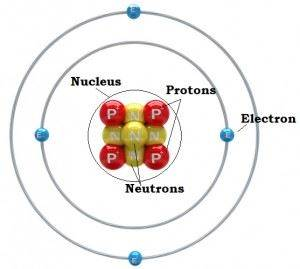FAQ on Atomic Structure in JEEIn the early nineteenth century, Dalton laid the foundation of the atomic structure. He explained the composition of matter and defined an atom as the smallest unit of matter that takes part in a chemical reaction. On the basis of the atomic theory, he explained the law of conservation of mass and law of constant composition. Atomic structure is the foundation of all the chemical reactions taking place. In JEE, the atomic structure holds a high weightage and is significant. The questions asked from this chapter are direct, mainly involving some basic concepts or formulae. It is less time consuming as compared to other topics. JEE (joint entrance examination) consists of three subjects namely, physics, chemistry and mathematics. In these subjects, chemistry is thought to be the most scoring and hence concern should first be given to chemistry. Questions asked in JEE generally follow a pattern and hence going through important topics at the last moment always helps.

Important Questions For Atomic Structure

Question 1: What are the important topics discussed in the atomic structure chapter that needs to be focused in order to crack JEE?

Answer: According to JEE syllabus the significant topics in the atomic structure are:

1. Discovery of electron, proton and neutron
2. Atomic models and Dalton’s atomic theory
4. Quantum mechanical model of an atom
5. Dual nature of matter and the photoelectric effect
6. Electronic configuration

The topic of atomic structure covers 5-7% marks in chemistry paper and these topics require an understanding of the concepts and retaining important formulae.

Question 2:  What are the types of questions that are generally asked?

Calculate:

(a) The de Broglie wavelength of an electron moving with a velocity of 5.0 × 105 ms–1.

(b) Relative de Broglie wavelength of an atom of hydrogen and atom of oxygen moving with the same velocity (h = 6.63 × 10–34 kg m2 s–1)

Solution:

(a)$\lambda$ = $\frac{h}{mv}$ = $\frac{6.63\times 10^{-34}\;kgm^2s^{-1}}{(9.11\;times\;10^{-31}\;kg)(5.0\;\times\;10^5\;ms^{-1})}$ = $1.46\; \times\;10^{-9}\;m$

Wavelength ($\lambda$) = $1.46 \;\times\;10^{-9}\;m$

(b) An atom of oxygen has approximately 16 times the mass of an atom of hydrogen. In the formula  $\lambda$ = $\frac{h}{mv}$  , h is constant while the condition of problem makes $v$, also constant. This means that $\lambda$ and m are variables and $\lambda$ varies inversely with m. Therefore, $\lambda$ for the hydrogen atom would be 16 times greater than $\lambda$ for oxygen atom.

Question 3: Which books should be referred for atomic structure while preparing for JEE?

Answer: Go through the NCERT chemistry textbook for class 11th, this book contains all the details in a very precise way. Physical Chemistry by O. P. Tandon is another good book which can be used as a reference. For numerical practice use the book Modern Approach to Chemical Calculations by R. C. Mukherjee.

For more details on JEE or this topic install Byju’s learning app.

Practise This Question

Weedicides are only poisonous to the unwanted plants growing in the farm land.